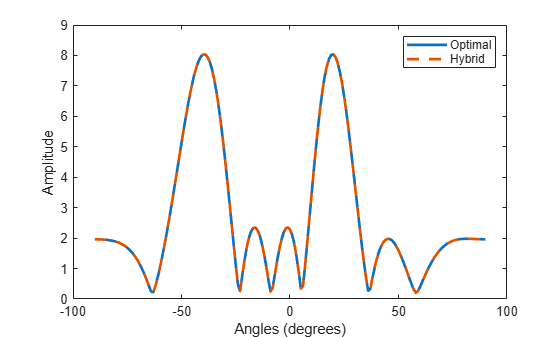# ompdecomp

Decompose signal using orthogonal matching pursuit

## Syntax

``[coeff,dictatom,atomidx,errnorm] = ompdecomp(X,dict)``
``[coeff,dictatom,atomidx,errnorm] = ompdecomp(X,dict,'MaxSparsity',nm)``
``[coeff,dictatom,atomidx,errnorm] = ompdecomp(X,dict,'NormWeight',wts)``

## Description

````[coeff,dictatom,atomidx,errnorm] = ompdecomp(X,dict)` computes the decomposition matrices `coeff` and `dictatom` of the signal `X`. The product of the decomposition matrices, `dictatom` x `coeff`, approximates `X`. The atoms in `dictatom` are selected from `dict`. `atomidx` are the indices in `dict` corresponding to `dictatom`. `errnorm` is the decomposition error. The decomposition is based on an orthogonal matching pursuit (OMP) algorithm that minimizes the Frobenius norm `||``X` – `dictatom` x `coeff``||`.```

example

````[coeff,dictatom,atomidx,errnorm] = ompdecomp(X,dict,'MaxSparsity',nm)` also specifies the maximum sparsity `nm`.```
````[coeff,dictatom,atomidx,errnorm] = ompdecomp(X,dict,'NormWeight',wts)` minimizes the weighted Frobenius norm `||``wts`1/2(`X` – `dictatom` x `coeff`)`||` using the weights `wts`.```

## Examples

collapse all

Given a set of optimal, full-digital, beamforming weights for an 8-element uniform linear array, decompose the weights into a product of analog and digital beamforming weights. Assume two RF chains. Show that the combined weights achieve a performance similar to the optimal weights.

Specify the optimal, full-digital, beamforming weights.

`wopt = steervec((0:7)*0.5,[20 -40]);`

Create a dictionary of steering vectors.

`stvdict = steervec((0:7)*0.5,-90:90);`

Perform the decomposition using OMP. Set the maximum sparsity to two.

`[wbb,wrf,wdictidx,normerr] = ompdecomp(wopt,stvdict,'MaxSparsity',2);`

Compare the beam patterns derived from the optimal weights and the hybrid weights. The plot shows that the decomposition of `wopt` into `wrf` and `wbb` is almost exact.

```plot(-90:90,abs(sum(wopt'*stvdict)),'-', ... -90:90,abs(sum((wrf*wbb)'*stvdict)),'--','LineWidth',2) xlabel('Angles (degrees)') ylabel('Amplitude') legend('Optimal','Hybrid')```## Input Arguments

collapse all

Input data to be decomposed, specified as a complex-valued N-by-Nc matrix.

Data Types: `double`
Complex Number Support: Yes

Dictionary of atoms, specified as a complex-valued matrix. The function uses a subset of atoms from the dictionary to construct the data.

Data Types: `double`
Complex Number Support: Yes

Maximum sparsity of the decomposition, specified as a positive integer. The decomposition stops when the sparsity of `nm` is achieved.

Example: `5`

#### Dependencies

Use this argument with the syntax specifying `'MaxSparsity'`.

Data Types: `double`

Norm weights used by OMP to minimize the weighted Frobenius norm of `||``wts`1/2 x (`X``dictatom` x `coeff`)`||`, specified as a complex-valued N-by-N matrix.

Example: `5`

#### Dependencies

Use this argument with the syntax specifying `'NormWeight'`.

Data Types: `double`
Complex Number Support: Yes

## Output Arguments

collapse all

Coefficients of basis atoms, returned as an Ns-by-Nc matrix. The rows represent the coefficients for the corresponding atoms in `dictatom`. Ns represents the number of atoms selected from the dictionary and is a measure of signal sparsity.

Data Types: `double`
Complex Number Support: Yes

Signal basis atoms, returned as an N-by-Ns matrix. The columns are the atoms forming the basis of the signal. These atoms are a subset of the dictionary specified in `dict`. Ns represents the number of selected atoms and is a measure of signal sparsity.

Data Types: `double`
Complex Number Support: Yes

Indices of the atoms selected from the dictionary `dict`, returned as a length-Ns row vector where `dict(:,atomidx) = dictatom`.

Data Types: `double`

Norm of the decomposition error, returned as a nonnegative scalar.

Data Types: `double`

In the context of hybrid beamforming, the `coeff` argument represents digital weights. `dictatom` represents analog weights and `dict` is a collection of steering vectors that can be used as analog weights.